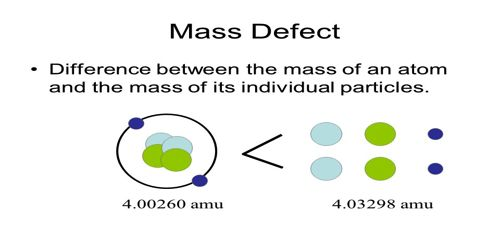# Mass Defect

Mass Defect

From the proton-neutron theory about the structure of the nucleus, we know that other than the hydrogen nucleus of atoms of all the elements are composed of proton and neutron. So, the mass of the nucleus should be equal to the masses of proton and neutrons. Mass Defect is the difference between the mass of an isotope and its mass number, representing binding energy. Proton and neutron are the ingredient particles of the nucleus. But the mass of a nucleus is found to differ from the sum of masses of proton and neutron. This difference in mass is known as a mass defect.

Suppose, the number of proton of a nucleus is Z and the number of neutrons is N. If the masses of protons and neutrons are respectively Mp and Mn, then the total mass of the nucleus is.

M = mass of protons + mass of neutrons,

or, M = ZMp + NMn

But, the mass of the permanent nucleus is found to be less than the combined mass of the constituent elements. This difference of the mass is called a mass defect.

It is generally expressed by Δm,

Mass defect, Δm = ZMp + NMn – M

or, Δm = ZMp + (A — Z)Mn – M

Here, A = mass number and N = (A — Z).

Now the question arises, where this missing mass goes? In answer, it can be said that during the formation of the nucleus this mass-has been radiated as energy and this energy is equal to the binding energy of the formation of the nucleus.